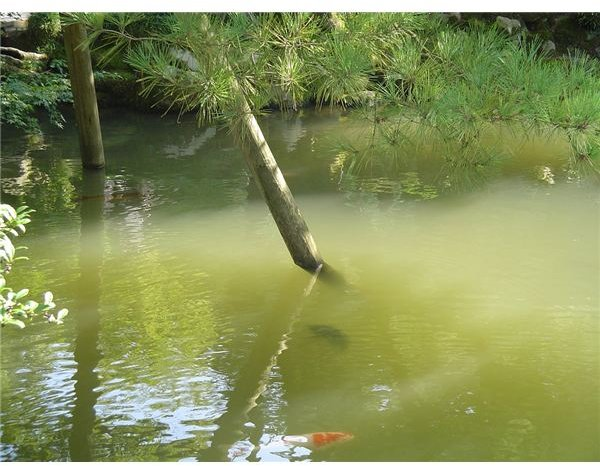# Converting & Comparing Numbers Written in Scientific Notation: Grade 8 Math LessonYou are a research biologist studying the life of microorganisms in pond water. You collected a sample of pond water in a jar. With an eye dropper, you placed one drop of the pond water on a microscope slide. You placed the slide under the lens of a microscope and observed the microorganisms. In your research study of the microorganisms living in pond water, you will use scientific notation to estimate the number of microorganisms living in the pond and the size of the microorganisms.

Lesson Objective: Lesson is aligned to the Common Core State Standards for Mathematics – 8.EE.3 Expressions and Equations – Use numbers expressed in the form of a single digit times an integer power of 10 to estimate very large or very small quantities, and to express how many times as much one is than the other. Lesson is aligned to the Common Core State Standards for Mathematics – 8.EE.4 Expressions and Equations – Perform operations with numbers expressed in scientific notation, including problems where both decimal and scientific notation are used. Use scientific notation and choose units of appropriate size for measurements of very large or very small quantities. Interpret scientific notation that has been generated by technology.

## Lesson Procedure

You have identified single-celled microorganisms attached to a plant as Stentors. You estimated the length of these microorganisms to measure between 0.002 and 0.003 m. Write the estimates of the lengths of the Stentors in scientific notation.

1. To write this number in scientific notation, move the decimal point so that the number lies between 1 and 10.
2. To write .002 in scientific notation, move the decimal point 3 places to the right.
3. To write very small numbers in scientific notation, negative integer exponents are used when the decimal point is moved to the right.
4. The small number 0.002 is written in scientific notation as 2 x 10-3. The exponent is -3 because the decimal point was moved 3 places to the right.
5. Write the small number 0.003 in scientific notation. Explain the steps you used to write the number in scientific notation.

Answer: 3 x 10-3. I moved the decimal point 3 places to the right so that the number is between 1 and 10. I used -3 as the exponent because I moved the decimal point to the right 3 places.

6. You found a second type of microorganism, the Cypris. You estimated the length of these microorganisms to measure between 0.0005 to 0.003 m. Write the estimates of the lengths of the Cypris in scientific notation.

Answer: 5 x 10-4 to 3 x 10-3

7. You estimated the length of one Stentor to be 2.5 x 10-3 and one Cypris to be 7.5 x 10-4. How much larger is the length of the Stentor to the length of the Cypris? Explain the steps you used to solve the problem.

Answer: 3 x 10-1 I divided 7.5 x 10-4 by 2.5 x 10-3. The number 7.5 divided by 2.5 equals 3. In a division problem, the exponents are subtracted. The exponent -4 (-) -3 equals -1.

## Downloads

Student Worksheet for Lesson 6

Student Assessment for the Unit

## This post is part of the series: Working with Radicals and Integer Exponents

This series of lessons is suitable for students in Grade 8. It covers working with positive and negative integer exponents and radicals. Students will be presented with real world scenarios and practice problems.